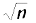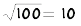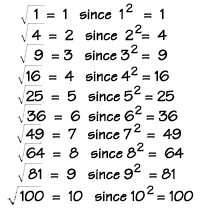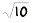Home    |    Teacher    |    Parents    |    Glossary    |    About UsMany mathematical operations have an inverse, or opposite, operation. Subtraction is the opposite of addition, division is the inverse of multiplication, and so on. Squaring, which we learned about in a previous lesson (exponents), has an inverse too, called "finding the square root." Remember, the square of a number is that number times itself. The perfect squares are the squares of the whole numbers: 1, 4, 9, 16, 25, 36, 49, 64, 81, 100 …

The square root of a number, n, writtenis the number that gives n when multiplied by itself. For example,because 10 x 10 = 100

Examples

Here are the square roots of all the perfect squares from 1 to 100.Finding square roots of of numbers that aren't perfect squares without a calculator

1. Estimate - first, get as close as you can by finding two perfect square roots your number is between.

2. Divide - divide your number by one of those square roots.

3. Average - take the average of the result of step 2 and the root.

4. Use the result of step 3 to repeat steps 2 and 3 until you have a number that is accurate enough for you.

Example: Calculate the square root of 10 () to 2 decimal places.

1. Find the two perfect square numbers it lies between.

Solution:
32 = 9 and 42 = 16, solies between 3 and 4.

2. Divide 10 by 3. 10/3 = 3.33 (you can round off your answer)

3. Average 3.33 and 3. (3.33 + 3)/2 = 3.1667

Repeat step 2: 10/3.1667 = 3.1579
Repeat step 3: Average 3.1579 and 3.1667. (3.1579 + 3.1667)/2 = 3.1623

Try the answer --> Is 3.1623 squared equal to 10? 3.1623 x 3.1623 = 10.0001

If this is accurate enough for you, you can stop! Otherwise, you can repeat steps 2 and 3.

Note: There are a number of ways to calculate square roots without a calculator. This is only one of them.

 Homework Help | Pre-Algebra | NumbersEmail this page to a friendSearch·  Place value·  Decimal numbers·  Estimating and     rounding·  Adding / subtracting     decimals·  Multiplying decimals·  Dividing decimals·  Percent·  Exponents·  Square roots·  Signed integers·  Adding and     subtracting integers·  Multiplying and     dividing integers·  Properties of integersFirst Glance In Depth Examples WorkoutSquare roots